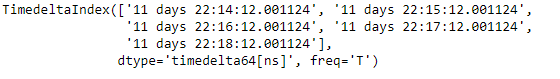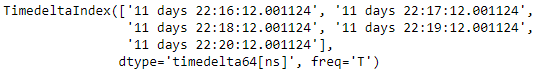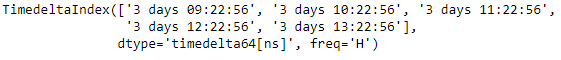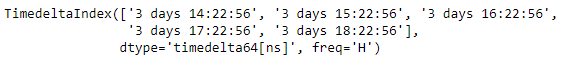# Python | Pandas TimedeltaIndex.shift()

• Last Updated : 01 Jan, 2019

Python is a great language for doing data analysis, primarily because of the fantastic ecosystem of data-centric python packages. Pandas is one of those packages and makes importing and analyzing data much easier.

Pandas` TimedeltaIndex.shift()` function performs specialized shift on the given TimedeltaIndex object, which produces a DatetimeIndex object.

Syntax : TimedeltaIndex.shift(n, freq=None)

Parameters :
n : Periods to shift by
freq : DateOffset or timedelta-like, optional

Return : shifted : DatetimeIndex

Example #1: Use `TimedeltaIndex.shift()` function to shift the given TimedeltaIndex object by 2 periods.

 `# importing pandas as pd``import` `pandas as pd`` ` `# Create the TimedeltaIndex object``tidx ``=` `pd.TimedeltaIndex(start ``=``'11 days 22:14:12.001124'``,``                                   ``periods ``=` `5``, freq ``=``'T'``)`` ` `# Print the TimedeltaIndex object``print``(tidx)`

Output :Now we will use the `TimedeltaIndex.shift()` function to shift each element of the given TimedeltaIndex object by 2 periods.

 `# shift by 2 periods``tidx.shift(n ``=` `2``)`

Output :As we can see in the output, the `TimedeltaIndex.shift()` function has returned a new object and it has shifted each element by 2 minutes.

Example #2: Use `TimedeltaIndex.shift()` function to shift the given TimedeltaIndex object by 2 periods.

 `# importing pandas as pd``import` `pandas as pd`` ` `# Create the TimedeltaIndex object``tidx ``=` `pd.TimedeltaIndex(start ``=``'03 days 09:22:56'``,``                            ``periods ``=` `5``, freq ``=``'H'``)`` ` `# Print the TimedeltaIndex object``print``(tidx)`

Output :Now we will use the `TimedeltaIndex.shift()` function to shift each element of the given TimedeltaIndex object by 5 periods.

 `# shift by 5 periods``tidx.shift(n ``=` `5``)`

Output :As we can see in the output, the `TimedeltaIndex.shift()` function has returned a new object and it has shifted each element by 5 hours.

My Personal Notes arrow_drop_up